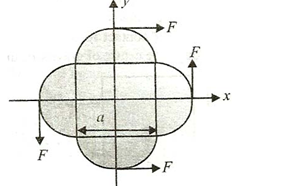# A planar object made up of a uniform square plate and four semicircular discs of the same thickness and material is being acted upon by four forces of equal magnitude as shown in Figure. The coordinates of point of application of forces is given bya)b)c)d)## Question ID - 100130 :- A planar object made up of a uniform square plate and four semicircular discs of the same thickness and material is being acted upon by four forces of equal magnitude as shown in Figure. The coordinates of point of application of forces is given bya)b)c)d)3537

(b)

The two forces along the- direction balance each other.Hence, the resultant force isalong the-direction. Let the point of application of the force be at ()

(By symmetry,-coordinate will be zero)

For rotational equilibrium:Alternative method:

Torque will only be produced by the two forces acting along-direction in anticlockwise direction. To balance this torque, we should apply a forcein order to produce a torque in the clockwise direction, which is only possible if we apply a force at a point below the-axis

ThenNext Question :

In the following question, numbers are arranged in a series with some principles understanding it.  One term in the series is missing as shown by _________.  This term is given as one of the alternatives amongst the four options given below it.  Find this term.

 9, 16, 25, 36, ___________, 64, 81 (a) 40 (b) 42 (c) 46 (d) 49